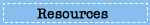# Sample Questions

## What questions are used in the NAEP mathematics assessment?

Explore sample questions from the mathematics assessment, and see how the NAEP mathematics questions relate to student performance.## Test Yourself in Mathematics

Select one of the grade tabs below to try out 10 questions from the 2009 NAEP mathematics assessment. After answering all the questions, compare your score with that of students nationally.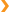Grade 8 Sample Questions

Question 1 of 10:

Marty has 6 red pencils, 4 green pencils, and 5 blue pencils. If he picks out one pencil without looking, what is the probability that the pencil he picks will be green?

1. 1 out of 3
2. 1 out of 4
3. 1 out of 15
4. 4 out of 15

Question 2 of 10:

The school carnival committee sold a total of 200 tickets for the grand prize drawing. Sue bought enough tickets so that she had a 20 percent chance of winning the grand prize. How many tickets did Sue buy?

1.      20
2.      40
3.    160
4.    400
5. 1,000

Question 3 of 10: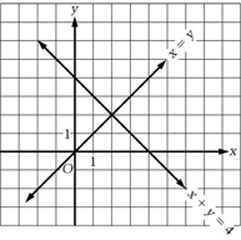Which point is the solution to both equations shown on the graph above?

1. (0, 0)
2. (0, 4)
3. (1, 1)
4. (2, 2)
5. (4, 0)

Question 4 of 10:

Megan drew a rectangle that has an area of 24 square centimeters. Which of the following could be the dimensions of her rectangle?

1.  2 centimeters by 12 centimeters
2.  3 centimeters by 9 centimeters
3.  4 centimeters by 20 centimeters
4.  6 centimeters by 6 centimeters
5. 12 centimeters by 12 centimeters

Question 5 of 10:

For a school report, Luke contacted a car dealership to collect data on recent sales. He asked, "What color do buyers choose most often for their car?" White was the response. What statistical measure does the response "white" represent?

1.  Mean
2.  Median
3.  Mode
4.  Range
5.  Interquartile range

Question 6 of 10: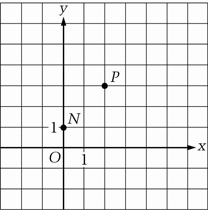For the figure above, which of the following points would be on the line that passes through points N and P?

1. (–2, 0)
2. (0, 0)
3. (1, 1)
4. (4, 5)
5. (5, 4)

Question 7 of 10:

The length of a rectangle is 3 feet less than twice the width, w (in feet). What is the length of the rectangle in terms of w ?

1. 3 – 2w
2. 2(w + 3)
3. 2(w – 3)
4. 2w + 3
5. 2w – 3

Question 8 of 10: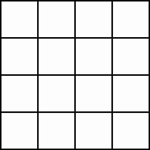Identical puzzle pieces have been put together to form the large square shown above. Which of the following could be the shape of each puzzle piece?

1.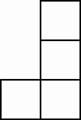2.

3.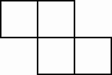4.

5.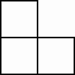6.

7.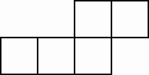8.

9.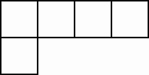Question 9 of 10: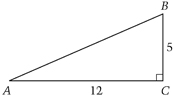In the right triangle above, what is the length of AB ?

1.   8.5
2. 12
3. 13
4. 17
5. 30

Question 10 of 10:

Which of the following is always an odd integer?

1. The product of two odd integers
2. The product of two consecutive integers
3. The sum of three even integers
4. The sum of two odd integers
5. The sum of three consecutive integersThe chart below shows the percentage of questions that you answered correctly, as well as how students at each of the achievement levels performed on this set of questions. For instance, eighth-grade students at Proficient had a percentage correct of 77% on average.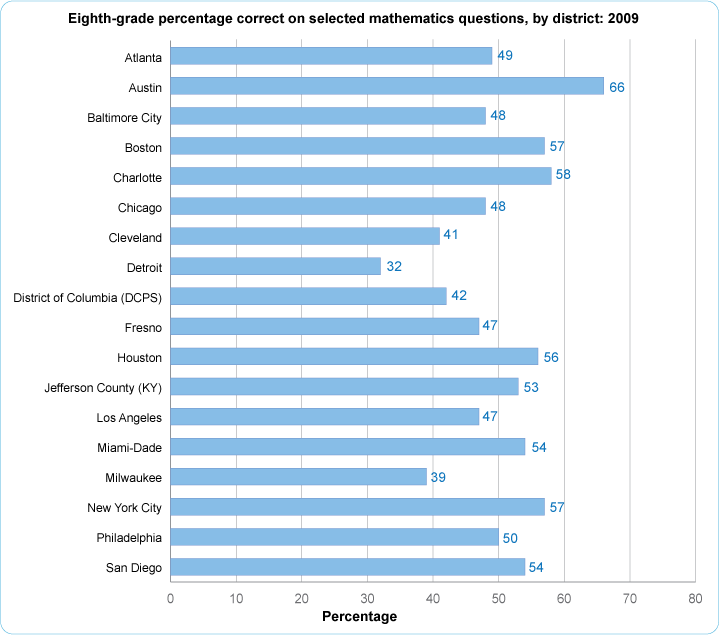•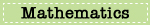•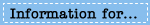•